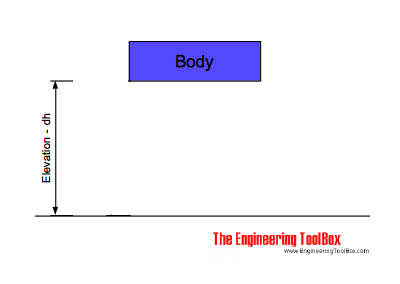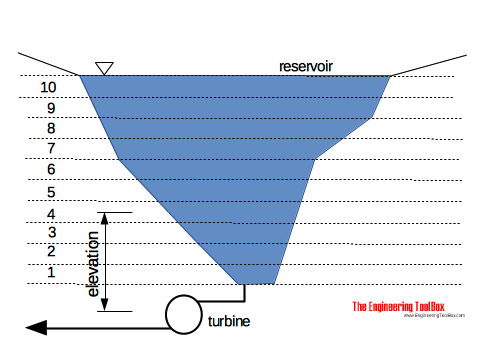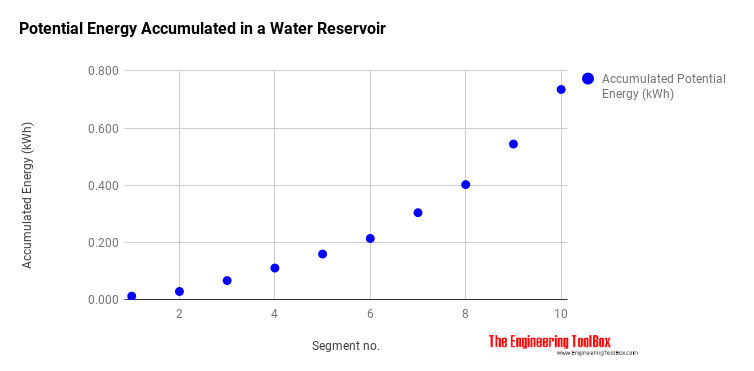Engineering ToolBox - Resources, Tools and Basic Information for Engineering and Design of Technical Applications!

# Potential Energy - Hydropower

## Elevation and potential energy in hydropower.

When a body of mass is elevated against the gravitational force - the increase in it's potential energy can be calculated as

Ep = Fg Δh

=  m ag Δh                                (1)

where

Fg = gravitational force (weight) acting on the body (N, lbf)

Ep = potential energy (J, ft lb)

m = mass of body (kg, slugs)

ag = acceleration of gravity on earth (9.81 m/s2, 32.17405 ft/s2)

Δh = change in elevation (m, ft)

### Example - Potential Energy of Elevated Body - in SI unitsA body of 1000 kg is elevated 10 m. The change in potential energy can be calculated as

Ep = (1000 kg) (9.81 m/s2) (10 m)

= 98100 J

= 98 kJ

= 0.027 kWh

### Example - Potential Energy of Elevated Body - in Imperial units

A body with weight (force) 500 lbf is elevated 30 ft. The change in potential energy can be calculated as

Ep = (500 lbf) (30 m)

= 15000 ft lb

A body with mass 15 slugs is elevated 30 ft. The change in potential energy can be calculated as

Ep = (15 slugs) (32.17405 ft/s2) (30 m)

= 14478 ft lb

### Potential Energy in a Tank or a Reservoir - Spreadsheet CalculatorYou can estimate potential elevation energy (hydropower) in a tank or a reservoir by dividing the volume in horizontal slices and calculate the elevation energy for each slice - as it is done in the spreadsheet calculator below:## Related Topics

• ### Dynamics

Motion - velocity and acceleration, forces and torque.
• ### Mechanics

Forces, acceleration, displacement, vectors, motion, momentum, energy of objects and more.

## Related Documents

• ### Energy

Energy is the capacity to do work.
• ### Energy Storage Density

Energy density - by weight and volume - for some ways to store energy
• ### Heat, Work and Energy

Heat vs. work vs. energy.
• ### Hydropower

Power potential vs. head and flow rate.
• ### Kinetic Energy

Energy possessed by an object's motion is kinetic energy.
• ### Mechanical Energy Equation vs. Bernoulli Equation

The Mechanical Energy Equation compared to the Extended Bernoulli Equation.
• ### Pressure Energy

Calculate the potential of pressure energy in a incompressible fluid.
• ### Pumps, Fans and Turbines - Horsepower

British Horse Power as used for pumps, fans and turbines - and how to convert to other units.
• ### Salt Hydrates - Melting points and Latent Melting Energy

Melting points and latent energy of salt hydrates.
• ### Sluice Gate - Volume Flow Measurements

Sluice gates can be used to control and measure volume flow rates in open channels and rivers, mainly in connection to hydro power plants.
• ### Static Pressure vs. Head

Static pressure vs. pressure head in fluids.
• ### Work done by Force

Work done by a force acting on an object.

## Engineering ToolBox - SketchUp Extension - Online 3D modeling!

Add standard and customized parametric components - like flange beams, lumbers, piping, stairs and more - to your Sketchup model with the Engineering ToolBox - SketchUp Extension - enabled for use with older versions of the amazing SketchUp Make and the newer "up to date" SketchUp Pro . Add the Engineering ToolBox extension to your SketchUp Make/Pro from the Extension Warehouse !

We don't collect information from our users. More about

## Citation

• The Engineering ToolBox (2008). Potential Energy - Hydropower. [online] Available at: https://www.engineeringtoolbox.com/potential-energy-d_1218.html [Accessed Day Month Year].

Modify the access date according your visit.

9.29.12